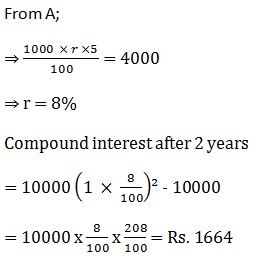New Students Offer - Use Code HELLO

# Data Sufficiency Test for SBI PO 2017Directions (1-5): Each of the questions below consists of a questions and three statements numbered A, B and C given below it. You have to decide whether the data provided in the statements are sufficient to answer the questions. Read both the statement and give the answer :

1. What is the present age of mother?
A. The difference in ages of mother and son is 30 years.
B. The present age of son is 1/4 present age of the mother.
C. After 5 years now, the age of son will be 1/3 of mother's age.

1. Only A and B
2. Only B and C
3. Only A and C
4. All A, B and C
5. Any two of the statement

2. What is the length of the train?
A. The train crosses a signal in 18 seconds.
B. The train crosses a 300 meter- long platform in 30 seconds.
C. The speed of the train is 25 km/hr

1. Only A and C
2. Only B and C
3. C and either A or B
4. Only A or B
5. Any two of the statements

3. What is the cost of fencing a rectangular park?
A. The length of the park is twice its breadth.
B. The area of the park is 288 m².
C. The Cost of fencing is Rs. 150 per meter.

1. Only B and C
2. Only A and C
3. All A, B and C
4. Only A and B
5. Cannot be determined

4. By selling and article, what is profit percentage gained?
A. 10% discount is given on marked price.
B. The article is marked 25% above the cost price.
C. The cost price of the article is Rs. 1000.

1. Only A and B
2. Only A and C
3. Only B and C
4. All A, B and C
5. Other than the given options

5. What will be the compound interest earned on an amount of Rs? 10000 in two years?
A. The SI on the same amount at the same rate of interest in five years is Rs. 4000.
B. The CI and SI earned in one year is the same.
C. The amount becomes more than double on Ci in 10 years.

1. Only A and B
2. Only A
3. Only B and C
4. Only A and C
5. Other than the given options

1. 5;
Let the mother's and son's present age of x and y years respectively
From A;  x-y = 30
From B; y = 1/4x
⇒ 4y = x
From C; y +  = 1/3 (x+5)
⇒ 3y + 15 = x + 5
⇒ x = 3y + 10
From A and B;
4y - y = 30
⇒ y = 30
∴ x = 40 years
From A and C;
30 + y = 3y + 10
⇒ 2y = 20
⇒ y = 10
∴ x = 40
From B and C;
4y - 3y = 10
⇒ y = 10
∴ x = 30 + 10 = 40
∴  We will get answer from any of the two statements.

2. 5;
Let the length of the train be x m and speed be v.Similarly we can find answer from 'A' and 'C' or from 'B' and 'C'.

3. 3;
From A; L = 2B
From B; L x B = 288
From C; Cost od fencing -= Rs. 150 per meter
From A, B and C; we get
2B x B = 288
B² = 144
∴ B = 12m
L = 24m
∴ Cost of fencing = 2 x (12+24) x 150 = Rs. 10,800

4. 4;
From A, B and C;
Cost price = Rs. 10005. 2;